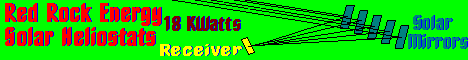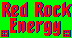FormulaeGo back to Red Rock Energy.
Mathamatical & Physical Formulae

pine1
Thermal Formulae

Nick Pine <nick_pine@verizon.net> wrote:

```cfm = 16.6Asqrt(HdT)                   (airflow up a chimney)
E = NTU/(NTU+1)                        (counterflow heat exch. efficiency)
E = (Tco-Tci)/(Thi-Tci)                (heat exchanger I/O temps)
E = (Thi-Tho)/(Thi-Tci)                (heat exchanger I/O temps)
NTU = AU/Cmin                          (number of heat transfer units)
Pa = 29.921/(1+0.62198/w)              (vapor pressure in air)
Pe = VIF                               (power in electical load)
Pe/A = 0.00509Vw^3                     (wind power density)
Ph = (Ti-To)U                          (Ohm's law for heatflow)
Ph = cfmdT                             (heat power carried by airstream)
Ph = 100A(Ps-Pa)                       (heat loss from uncovered pool)
Ph = 0.1714x10^-8Ae(Th^4-Tc^4)         (radiation heat loss)
Ps = exp(17.863-9621/(460+T))          (vapor pressure at 100% RH)
R = Rv/A = d/(kA)                      (thermal resistance of a wall)
RH = 100Pa/Pw                          (relative humidity)
Rv = d/k                               (US "R-value")
Q = C(Th-Tc)                           (stored heat energy)
Trc = Tc+460                           (absolute temp of cold object)
Trdp = Trc/(1+Trcln(RH/100)/9621)      (absolute dew point)
Trh = Th+460                           (absolute temp of hot object)
T(t) = Tf+(Tf-Ti)exp(-t/RC)            (temp after t hours in Tf air)
Trwb = 9621/(22.47+ln(100Pa+Tra-Twrb)) (abs. wet bulb temp, iterate)
U = kA/d = A/Rv                        (thermal conductance of a wall)

Definitions:

A    area (ft^2)
C    heat capacitance (Btu/F)
cfm  airflow (ft^3/min)
Cmin heat capacity flow rate (Btu/h-F)
d    thickness (inches)
dT   temp diff (F)
e    emissivity of surface (shiny => 0, dull => 1)
E    heat exchanger efficiency
F    power factor (DC =>1)
H    height difference (ft)
I    current (A)
k    thermal conductivity (Btu-in/h-F-ft^2)
P    power (watts)
Pa   water vapor pressure in air ("Hg)
Pe   heat power (Btu/h)
Pw   water vapor pressure near pool ("Hg)
Q    heat energy (Btu)
R    thermal resistance (F-h/Btu)
RC   time constant (hours)
RH   relative humidity (%)
Rv   US "R-value" in ft^2-F-h/Btu
t    time delay (hours)
T    temperature (F)
Tc   cold temperature (F)
Tci  cold fluid temp entering heat exchanger (F)
Tco  cold fluid temp leaving heat exchanger (F)
Trdp absolute dew point (R)
Tf   final temp (F)
Thi  hot fluid temp entering heat exchanger (F)
Tho  hot fluid temp leaving heat exchanger (F)
Ti   initial temp (F)
Trc  cold absolute temperature (R)
Th   hot temperature (F)
Trh  hot absolute temperature (R)
Trwb absolute wet bulb temp (R)
U    thermal conductance (Btu/h-F)
V    voltage (V)
Vw   windspeed (mph)
w    humidity ratio (#water/#dry air)

Conductivities (k, in Btu-in/h-F-ft^2):

still air, 0.18; Styrofoam, 0.2; water,
4.2 (for downward heatflow); ice, 1.1;
concrete, 5; soil, 5-15; glass, 7;
steel, 314; copper, 2724

R-values (Rv in Btu/h-F-ft^2):

slow-moving air, 2/3; single-pane window, 1;
double-pane, 2;
double foil with large airspaces & downward heatflow, 10

Densities (lb/ft^3):

air, 0.075; ice, 57.2; water, 62.3;
concrete, 150; steel, 450

Specific heats by volume (Btu/F-ft^3):

air, 0.02; concrete, 25; ice, 28; steel, 59; water, 63

Latent heats (Btu/lb):

ice melts, 144; water evaporates, 970

Metric equivalents:

1 m = 3.281 ft    1 m/s = 2.24 mph        1 kg = 2.205 lb
1 kJ = 0.948 Btu  1 kWh = 3412 Btu        1 kW = 1.341 HP
1 L = 0.264 gal   Rvm = Rv/5.68 (W/m^2C)  deg C = 5/9(deg F-32)

Long-term average Phila weather:

Jan       July

daily min temp     22.8      67.2    F
24-h avg           30.4      76.7    F
daily max          37.9      86.1    F
sun on S wall    1000       820      Btu/ft^2
E/W  "      425      1030      Btu/ft^2
N    "      190       580      Btu/ft^2
horiz surf    620      1890      Btu/ft^2
humidity ratio w    0.0025    0.0133
windspeed          10.3       8.0    mph

Betz (wind efficiency) limit: 0.593```

<redrok@redrok.com>